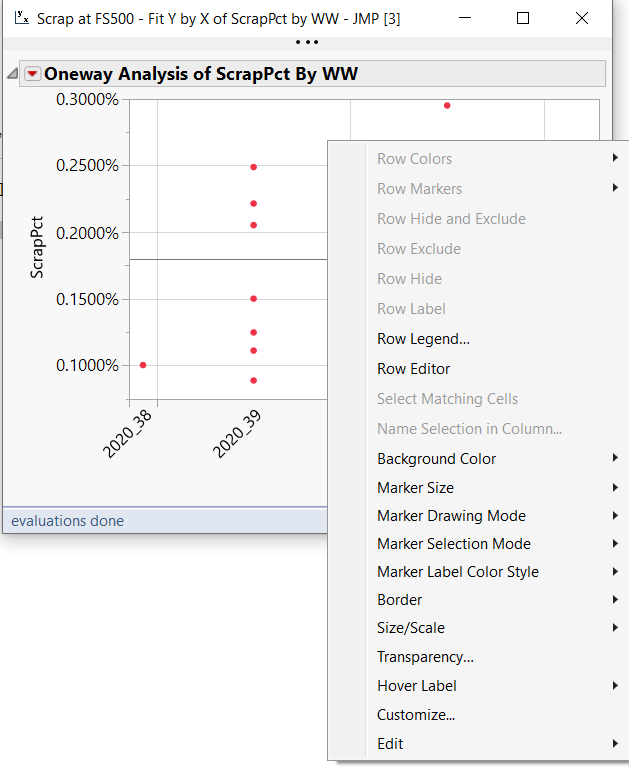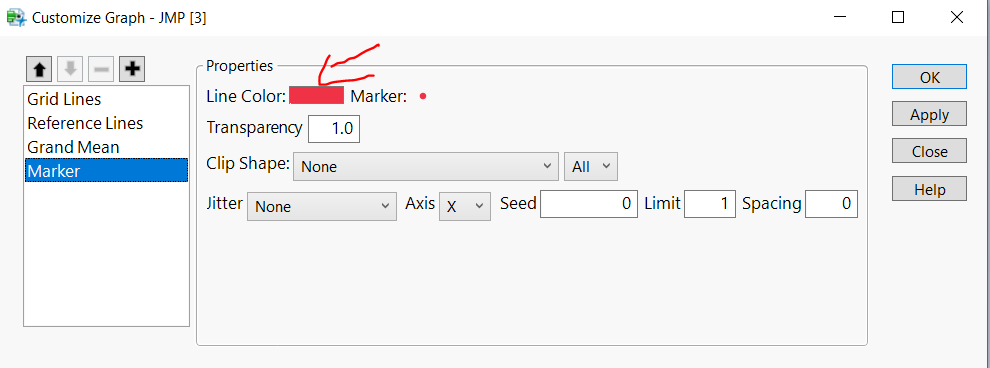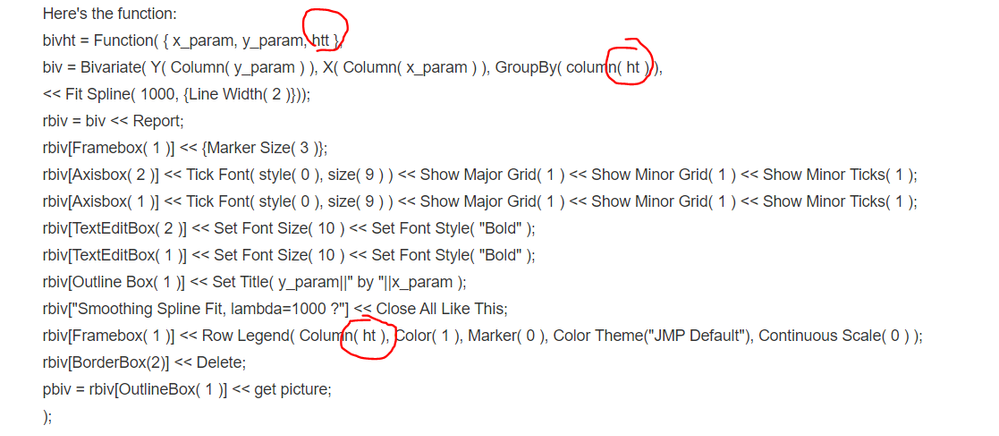Our World Statistics Day conversations have been a great reminder of how much statistics can inform our lives. Do you have an example of how statistics has made a difference in your life? Share your story with the Community!
Choose Language Hide Translation Bar
Highlighted

## Fit Y by X Spline fit color

Hi,

I have a fit Y by X plot with smoothing spline fit, is there any way that I can make the marker colors gray and the spline fit line in JMP default color? I tried changing the color theme into medium light gray, but it's not working

``rbiv[Framebox( 1 )] << Row Legend( Column( col1 ), Color( 1 ), Marker( 0 ), Color Theme("JMP Default"), Continuous Scale( 0 ) );``
10 REPLIES 10
Highlighted

## Re: Fit Y by X Spline fit color

You can right click on the graph and select "Customize"then select the color you wantThen export the script to window to see the real code behind

``````Oneway(
Y( :Yield ),
X( :WW ),
SendToReport(
Dispatch(
{},
"Oneway Plot",
FrameBox,
{DispatchSeg(
Marker Seg( 1 ),
{Color( "Orange" ), Marker( "Triangle" )}
)}
)
)
);``````
Highlighted

## Re: Fit Y by X Spline fit color

Hi, I tried this method but it is not working when I put it into my function
Highlighted

## Re: Fit Y by X Spline fit color

Can you share your full code?
Highlighted

## Re: Fit Y by X Spline fit color

Here's the function:

``````bivht = Function( {x_param, y_param, htt},
biv = Bivariate(
Y( Column( y_param ) ),
X( Column( x_param ) ),
GroupBy( Column( ht ) ),
<<Fit Spline( 1000, {Line Width( 2 )} )
);
rbiv = biv << Report;
rbiv[Framebox( 1 )] << {Marker Size( 3 )};
rbiv[Axisbox( 2 )] << Tick Font( style( 0 ), size( 9 ) ) << Show Major Grid( 1 ) << Show Minor Grid( 1 ) <<
Show Minor Ticks( 1 );
rbiv[Axisbox( 1 )] << Tick Font( style( 0 ), size( 9 ) ) << Show Major Grid( 1 ) << Show Minor Grid( 1 ) <<
Show Minor Ticks( 1 );
rbiv[Text Edit Box( 2 )] << Set Font Size( 10 ) << Set Font Style( "Bold" );
rbiv[Text Edit Box( 1 )] << Set Font Size( 10 ) << Set Font Style( "Bold" );
rbiv[Outline Box( 1 )] << Set Title( y_param || " by " || x_param );
rbiv["Smoothing Spline Fit, lambda=1000 ?"] << Close All Like This;
rbiv[Framebox( 1 )] << Row Legend(
Column( ht ),
Color( 1 ),
Marker( 0 ),
Color Theme( "JMP Default" ),
Continuous Scale( 0 )
);
rbiv[Border Box( 2 )] << Delete;
pbiv = rbiv[Outline Box( 1 )] << get picture;
);``````
Highlighted

## Re: Fit Y by X Spline fit color

Is this a typo or you have another variable named "ht"Highlighted

## Re: Fit Y by X Spline fit color

ht is a variable name
Highlighted

## Re: Fit Y by X Spline fit color

I mean in your function, you define htt but you never use it. Instead, you use ht
That could be the issue
Highlighted

## Re: Fit Y by X Spline fit color

``````lb = Lineup Box( N Col( 4 ) );
For( j = 1, j <= N Items( xparam ), j++,
bivht( xparam[j], yparam[j], h );
lb << append( pbiv );
biv << Close Window;
);
ppt9 << append( lb << get picture );``````
Highlighted
Article Labels